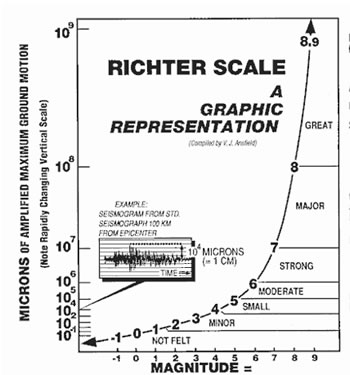# How much more powerful is a 7.0 earthquake than a 6.0?

Aug 29, 2015

A 7.0 earthquake is 10 times more powerful than a 6.0 earthquake.

#### Explanation:

The magnitude of an earthquake is measured by the Richter scale.

This is a logarithmic scale in which the magnitude is defined as the logarithm of the ratio of the amplitude of the seismic waves to an arbitrary minor amplitude.

If you are interested in the math, here's how it works.

For earthquakes of two different magnitudes $M$ with corresponding amplitudes $A$,

${M}_{2} - {M}_{1} = {\log}_{10} \left({A}_{2} / {A}_{1}\right)$

If the two magnitudes are 7.0 and 6.0,

log_10(A_2/A_1) = M_2 – M_1 = 7.0-6.0=1.0

${A}_{2} / {A}_{1} = {10}^{1.0} = 10$ or ${A}_{2} = 10 {A}_{1}$

The shaking amplitude of a 7.0 earthquake is 10 times that of a 6.0 earthquake.

If you aren't interested in the math, just remember that a difference of one unit in magnitude corresponds to a 10-fold increase in shaking amplitude.

Here is a graphic representation of the Richter scale.Note the exponential scale for the vertical axis.